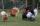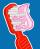Wine

A bottle of wine costs 21 euros; and wine is 20 times more expensive than a bottle. How much a bottle cost?

Result

f =  1 Eur

Solution:Leave us a comment of this math problem and its solution (i.e. if it is still somewhat unclear...):Be the first to comment!To solve this verbal math problem are needed these knowledge from mathematics:

Do you have a linear equation or system of equations and looking for its solution? Or do you have quadratic equation?

Next similar math problems:

1. BirdsOn the farm they have a total of 110 birds. Geese and turkeys together is 47. Hens is three times more than the turkey. How much is poultry by species?
2. One frame5 picture frames cost € 12 more than three frames. How much cost one frame?
3. Apples 3Julka has 5 apples more than Hugo and four apples less than Annie. Hugo has 17 apples. How many apples has Julka and how Annie?
4. BenchesThe park has 64 benches. Occupied are by 18 more than empty. How many benches are occupied and empty ?
5. Chewing gumsFor 3 chewing gums, you will pay 20 CZK less than 7 chewing gums. How much is 1 chewing gum and how much does a 5pcs package cost?
6. ExpressionSolve for a specified variable: P=a+4b+3c, for a
7. Addition of Roman numbersAdded together and write as decimal number: LXVII + MLXIV
8. Six te 2If 3t-7=5t, then 6t=
9. Forest nurseryIn the forest nursery after winter, they found that 1/10 stems died out of them. For them, they land 193 new spruces. How many spruces are in the forest nursery?
10. SimplifySimplify the following problem and express as a decimal: 5.68-[5-(2.69+5.65-3.89) /0.5]
11. Eq1Solve equation: 4(a-3)=3(2a-5)
12. If-then equationIf 5x - 17 = -x + 7, then x =
13. Simple equation 8Solve the following equation: 36=-(1+7x)-6(-7-x)
14. CagesHonza had three cages (black, silver, gold) and three animals (guinea pig, rat and puppy). There was one animal in each cage. The golden cage stood to the left of the black cage. The silver cage stood on the right of the guinea pig cage. The rat was in the
15. PopsiclesFrancis went to buy ice lollies. If he buy 8 popsicles he missed 4 USD. When he buy 7 popsicles, got back 1 USD. How many USD was a popsicle?
16. Foot in busIt was 102 people on the bus. 28 girls had two dogs. A 11 girls had one dog. At the next stop seceded 5 dogs (even with their owners). They got two boys together with three dogs. The bus drove one driver. How many foot were in bus?
17. Pets in ZOOThe zoo have run for pets. They are chickens, pigs and cow. Altogether there has 20 heads and 60 feet. How many chickens and piglets are there?